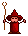###Author Topic: shortest distance between two lines (2D & 3D)  (Read 4916 times)

####r0ber7

• Prof. Inline
••• Posts: 552##### shortest distance between two lines (2D & 3D)
« on: 2013-Jul-12 »
While coding my physics engine, I found I needed an algorithm to calculate the closest distance between two lines. After some searching I found this, which I've converted into GLBasic.

This code will work in 2D and 3D. For 2D calculations, just enter zero for all z values.Code: (glbasic) [Select]
`TYPE point    x    y    zENDTYPE TYPE geo_line     x1     y1     z1     x2     y2     z2ENDTYPETYPE geometry///*//* //* http://softsurfer.com/Archive/algorithm_0106/algorithm_0106.htm//*//* Original C++ Copyright Notice//* ===================//* Copyright 2001, softSurfer (www.softsurfer.com)//* this code may be freely used AND modified FOR any purpose//* providing that this copyright notice is included with it.//* SoftSurfer makes no warranty FOR this code, AND cannot be held//* liable FOR any real OR imagined damage resulting from its use.//* Users of this code must verify correctness FOR their application.//*/FUNCTION norm : c1 AS point LOCAL nrm = SQR(dot(c1, c1)) //console.out("geometry.norm> "+nrm+"\n")    RETURN nrmENDFUNCTIONFUNCTION dot : c1 AS point, c2 AS point LOCAL dp = (c1.x * c2.x + c1.y * c2.y + c1.z * c2.z) //console.out("geometry.dot> "+dp+"\n")    RETURN dpENDFUNCTION FUNCTION linesegments_shortest_distance : line1 AS geo_line, line2 AS geo_line    LOCAL EPS = 0.00000001     LOCAL delta21 AS point    delta21.x = line1.x2 - line1.x1    delta21.y = line1.y2 - line1.y1    delta21.x = line1.z2 - line1.z1     LOCAL delta41 AS point    delta41.x = line2.x2 - line2.x1    delta41.y = line2.y2 - line2.y1    delta41.z = line2.z2 - line2.z1     LOCAL delta13 AS point    delta13.x = line1.x1 - line2.x1    delta13.y = line1.y1 - line2.y1    delta13.z = line1.z1 - line2.z1     LOCAL a = dot(delta21, delta21)    LOCAL b = dot(delta21, delta41)    LOCAL c = dot(delta41, delta41)    LOCAL d = dot(delta21, delta13)    LOCAL e = dot(delta41, delta13)    LOCAL D = a * c - b * b      LOCAL sc, sN, sD = D     LOCAL tc, tN, tD = D     IF D < EPS            sN = 0.0        sD = 1.0        tN = e        tD = c        ELSE            sN = (b * e - c * d)        tN = (a * e - b * d)        IF sN < 0.0                    sN = 0.0            tN = e            tD = c                ELSEIF sN > sD                    sN = sD            tN = e + b            tD = c        ENDIF    ENDIF     IF tN < 0.0            tN = 0.0         IF -d < 0.0            sN = 0.0        ELSEIF -d > a            sN = sD        ELSE                    sN = -d            sD = a        ENDIF    ELSEIF tN > tD        tN = tD        IF (-d + b) < 0.0            sN = 0        ELSEIF (-d + b) > a            sN = sD        ELSE                    sN = (-d + b)            sD = a        ENDIF    ENDIF     IF ABS(sN) < EPS     sc = 0.0    ELSE     sc = sN / sD    ENDIF        IF ABS(tN) < EPS    tc = 0.0    ELSE     tc = tN / tD    ENDIF     LOCAL dP AS point    dP.x = delta13.x + (sc * delta21.x) - (tc * delta41.x)    dP.y = delta13.y + (sc * delta21.y) - (tc * delta41.y)    dP.z = delta13.z + (sc * delta21.z) - (tc * delta41.z)   // shortest distance between two line segments   LOCAL sdist = SQR(dot(dP, dP))  //console.out("geometry.linesegments_shortest_distance> "+sdist+"\n")    RETURN sdist    ENDFUNCTION`
« Last Edit: 2014-May-03 by Moru »

####bigsofty

• Community Developer
• Prof. Inline
••• Posts: 2717##### Re: shortest distance between two lines (2D & 3D)
« Reply #1 on: 2013-Jul-13 »
Many thanks!Cheers,

Ian.

“It is practically impossible to teach good programming style to students that have had prior exposure to BASIC.  As potential programmers, they are mentally mutilated beyond hope of regeneration.”
(E. W. Dijkstra)